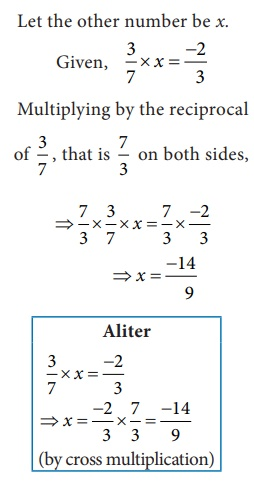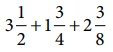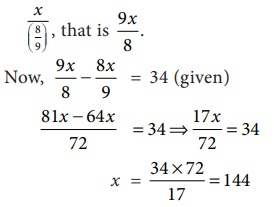Home | | Maths 8th Std | Word Problems on the basic operations

# Word Problems on the basic operations

Word Problems on the basic operations : Example Questions with Answers, Solution

Word Problems on the basic operations

Example 1.16

The sum of two rational numbers is 4/5 . If one number is 2/15, then find the other.

Solution:

Let the other number be x.Example 1.17

The product of two rational numbers is −2/3, If one number is 3/7, then find the other.

Solution:

Let the other number be x.Example 1.18

One roll of ribbon is 18 3/4 m long. Sankari has four full rolls and one–third of another roll. How many metres of ribbon does Sankari have in total?

Solution:

Number of metres of ribbon Sankari has in totalExample 1.19

Find the rational numbers that should be added and subtracted so that they will make the sumto the nearest whole number.

Solution:which lies between the whole numbers 7 and 8.

If we subtract 5/8 from 7 5/8 , it becomes 7. If we add 3/8 to 7 5/8, it becomes 7+ 5/8 + 3/8 = 7+1=8.

Example 1.20

A student instead of multiplying a number by 8/9, by mistake divided it by 8/9 . If the difference between the correct answer and the answer got by him is 34, then find the number.

Solution:

Let the number be x .Example 1.21Tags : Numbers | Chapter 1 | 8th Maths , 8th Maths : Chapter 1 : Numbers
Study Material, Lecturing Notes, Assignment, Reference, Wiki description explanation, brief detail
8th Maths : Chapter 1 : Numbers : Word Problems on the basic operations | Numbers | Chapter 1 | 8th Maths